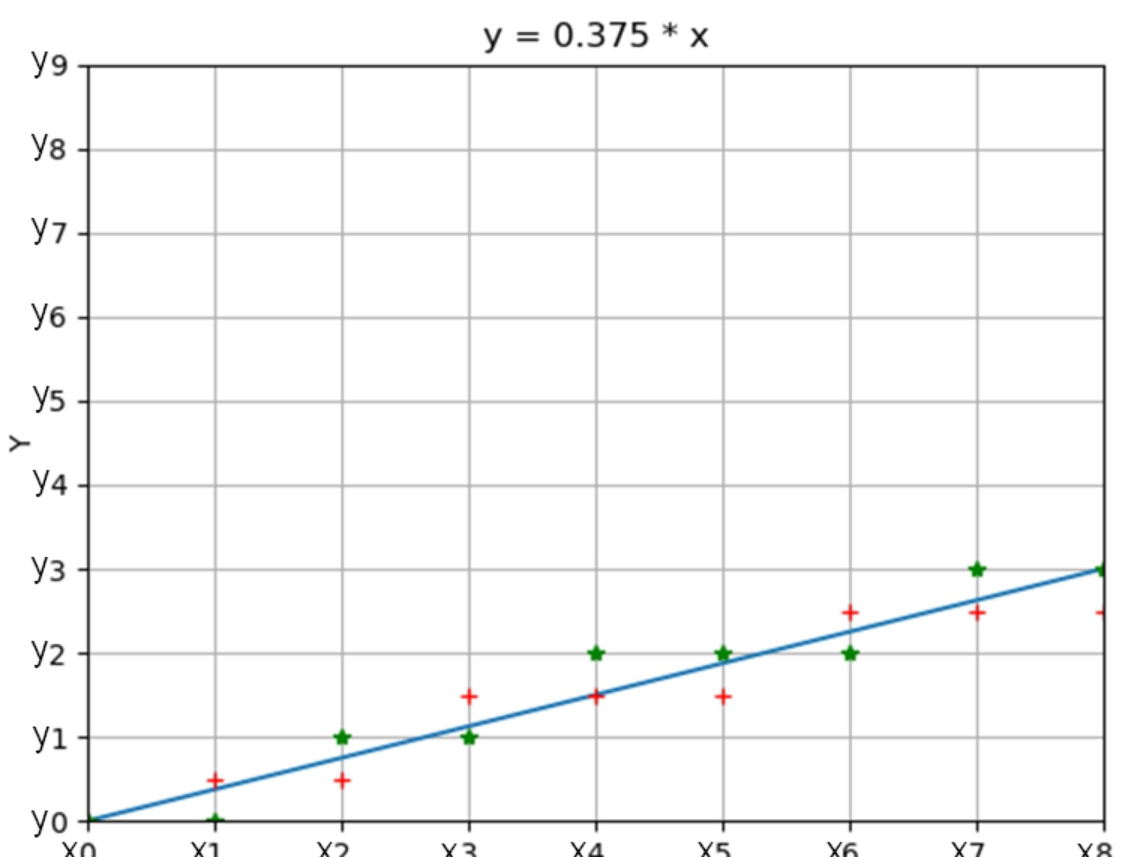## 基本原理## 一、算法1思路

1. 根据两点坐标算出斜率k=dy/dx（通常为浮点数）
2. 斜线与x=x1的交点y坐标y=x1+k ->往后自增k
3. y0、y1中点y坐标 middle=x1+0.5 ->往后根据两者位置增1或不变
4. 判别式：y>=middle
参考代码：
``````void OLED_ShowLine(u8 x1,u8 y1,u8 x2,u8 y2)
{

u8 dx = x2 - x1;
u8 dy = y2 - y1;
float k = (float)(dy) / dx;
u8 x = x1 + 1;		    //直线与x=x1的交点的x坐标
float y = k;	        //直线与x=x1的交点的y坐标,由于在判别式中y1相消，可以去掉y1
float middle = 0.5;     //第一个中点，由于在判别式中y1相消，可以去掉y1

OLED_DrawPoint(x1,y1,1);//画起点
OLED_DrawPoint(x2,y2,1);//画终点

while( x < x2 )			//中间线段
{

if(y >= middle)		//若直线点在中点或之上
{

y1++;
middle++;
}
OLED_DrawPoint(x,y1,1);//画点
x++;
y += k;
}
}
``````

## 二、算法2思路

1. 针对算法1解决浮点数问题，改用整数运算，方法：同比例放大法
2. 针对判别式修改：k>0.5，即dy/dx>0.5，两边乘以2倍dx，得2dy>dx
3. 此时斜线与x=x1的交点y坐标y=2dy ->往后自增2dy
4. y0、y1中点y坐标 middle=dx ->往后根据两者位置增2dx或不变
参考代码：
``````void OLED_ShowLine(u8 x1,u8 y1,u8 x2,u8 y2)
{

u8 dx = x2 - x1;
u8 dy = y2 - y1;
u8 x = x1 + 1;		    //直线与x=x1的交点的x坐标
u16 y = (dy<<1);	        //直线与x=x1的交点的y坐标,由于在判别式中y1相消，可以去掉y1
u16 middle = dx;     //第一个中点，由于在判别式中y1相消，可以去掉y1

OLED_DrawPoint(x1,y1,1);//画起点
OLED_DrawPoint(x2,y2,1);//画终点

//y=dy/dx + dy/dx  >=  middle=0.5 + 1

while( x < x2 )			//中间线段
{

if(y >= middle)		//若直线点在中点或之上
{

y1++;
middle+=(dx<<1);
}
OLED_DrawPoint(x,y1,1);//画点
x++;
y += (dy<<1);
}
}
``````

## 三、算法3思路

1. 针对算法2解决斜率和其他象限不可用问题
2. 引入坐标轴变换，保证dx为最长轴，交换后标记
3. 引入sign_x及sign_y标记符，当起始点大于终点则signe_x或sign_y为-1
参考代码：
``````void OLED_ShowLine(u8 x1,u8 y1,u8 x2,u8 y2)
{

u8 i=0,temp=0;
u8 interChange = 0;
u8 dx=0,dy=0;
int sign_x=0,sign_y=0;
u8 x = 0;
u16 y = 0;
u16 middle = 0;

//取绝对值，并标记
x2-x1>=0?(dx=x2-x1,sign_x=1):(dx=x1-x2,sign_x=-1);
y2-y1>=0?(dy=y2-y1,sign_y=1):(dy=y1-y2,sign_y=-1);

if(dx<dy)				//交换坐标轴,保证dx为长轴
{

temp = dx;
dx = dy;
dy = temp;
interChange = 1;	//标记
}

x = x1;            		//直线与x=x1的交点的x坐标
y = (dy<<1);       		//直线与x=x1的交点的y坐标,由于在判别式中y1相消，可以去掉y1
middle = dx;       		//第一个中点，由于在判别式中y1相消，可以去掉y1

OLED_DrawPoint(x1,y1,1);//画起点
OLED_DrawPoint(x2,y2,1);//画终点

for(i=0;i<(dx-1);i++)	//中间线段
{

if(interChange==0)
{

x+=sign_x;
}else{

y1+=sign_y;
}

if(y > middle)		//若直线点在中点或之上
{

if(interChange==0)
y1+=sign_y;
else
x+=sign_x;
middle+=(dx<<1);
}

OLED_DrawPoint(x,y1,1);//画点

y += (dy<<1);
}
}

``````

### 【bzoj2938】[Poi2000]病毒 AC自动机-程序员宅基地

Description二进制病毒审查委员会最近发现了如下的规律：某些确定的二进制串是病毒的代码。如果某段代码中不存在任何一段病毒代码，那么我们就称这段代码是安全的。现在委员会已经找出了所有的病毒代码段，试问，是否存在一个无限长的安全的二进制代码。 示例： 例如如果{011, 11, 00000}为病毒代码段，那么一个可能的无限长安全代码就是010101…。如果{01, 11, 000000}为病

### C++11 新特性总结_-std=c++11-程序员宅基地

【0】g++/gcc 支持c++11/c11标准{(0)为了让g++能够支持和编译c++11新特性代码，需在编译时候加上指定条件：-std=c++11g++ newFeature.cpp -o app -std=c++11(1)使用-std=c11来让gcc支持c11新特性gcc 文件名.c -o 可执行文件名 -std=c11}【1】静态断言（static_assert）: #include <assert.h>{(1)断言概念     所谓“断..._-std=c++11

### 用switch...case语句统计数字、空格和其他字符出现的次数-程序员宅基地

//用switch...case语句统计数字、空格和其他字符出现的次数//转自K&R#include int main(void) { int c, i, nwhite, nother, ndigit; nwhite = nother

### python画函数求交点_python3数学建模基础（四）多个函数图像求交点-程序员宅基地

python3数学建模基础(四)多个函数图像求交点,多个,交点,生姜,建模,函数python3数学建模基础(四)多个函数图像求交点python3数学建模基础(四)多个函数图像求交点本文以sin(x)和e^(-x)为例，用python3实现数学建模中多个函数求交点#作者#生姜用户import numpy as npimport mathfrom matplotlib import pyplot as..._python 求两个函数的交点

### PKCS #8 / OpenSSL Encrypted Keys-程序员宅基地

Commons-SSL includes support for extracting private keys from PKCS #8 files. We also support the OpenSSL formats ("traditional SSLeay"). The private keys can be in PEM (base64) or DER (raw ASN.1 - a b

### mysql ds.xml_ejb3.0数据源配置文件mysql-ds.xml oracle-ds.xml persistence.xml sqlserver-ds.xml_知乎视频的博客-程序员宅基地

ejb3.0数据源配置文件，直接COPY后将数据库名、用户名、密码改成你自己的，就能使用了。具体如下：mysql-ds.xml ：DefaultMySqlDSjdbc:mysql://localhost:3306/crud?useUnicode=true&characterEncoding=GBKorg.gjt.mm.mysql.Driverrootroot332org.jboss.res...